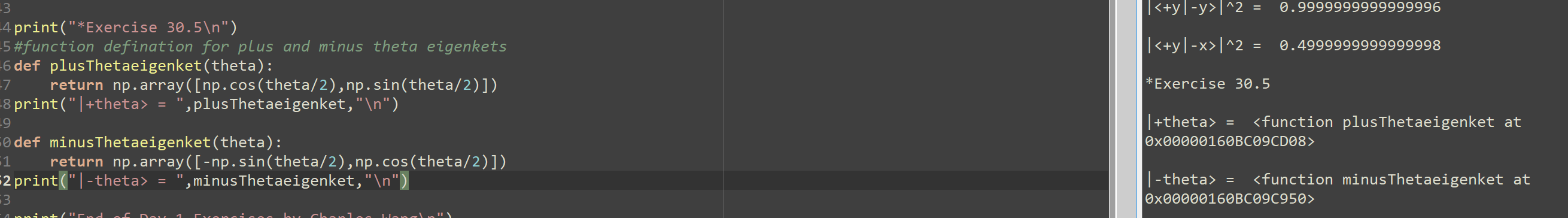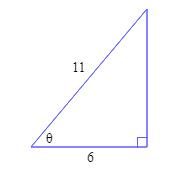# Solve each equation for o is less than and/or equal to theta is less than and/or equal to 360 -- sin^2x = 1 = cos^2x -- Work: cos^2x - cos^2x = 0 0 = 0 -- Textbook Answers: 90 and 270 -- Btw, how would you isolate for cos^2x = 0 related homework questions

• #### Solve each equation for o is less than and/or equal to theta is less than and/or equal to 360 -- sin^2x = 1 = cos^2x -- Work: cos^2x - cos^2x = 0 0 = 0 -- Textbook Answers: 90 and 270 -- Btw, how would you isolate for cos^2x = 0

Solve each equation for o is less than and/or equal to theta is less than and/or equal to 360--sin^2x = 1 = cos^2x--Work:cos^2x - cos^2x = 00 = 0--Textbook Answers:90 and 270--Btw, how would you isolate for cos^2x = 0? Would it be...x = cos^-1 square root of _____ ?

• #### sin^2 theta + 2 cos theta -1/ (sin^2 theta +3 cos theta-3= cos^2 theta+cos theta/ (-sin^2 theta) Prove the identity

sin^2 theta + 2 cos theta -1/ (sin^2 theta +3 cos theta-3= cos^2 theta+cos theta/ (-sin^2 theta)Prove the identity.

• #### def plusThetaeigenket(theta): return np.array([np.cos(theta/2),np.sin(theta/2)]) print("|+theta> = ",plusThetaeigenket,"\n") def minusThetaeigenket(theta): return np.array([-np.sin(theta/2),np.cos(theta/2)]) print("|-theta> = ",minusThetaeigenket,"\def plusThetaeigenket(theta): return np.array([np.cos(theta/2),np.sin(theta/2)]) print("|+theta> = ",plusThetaeigenket,"\n") def minusThetaeigenket(theta): return np.array([-np.sin(theta/2),np.cos(theta/2)]) print("|-theta> = ",minusThetaeigenket,"\n") Can you please help to solve where this python code went wrong for display the correct eigen ket value for theta? Thank you |<+y|-y>l^2 = 0.9999999999999996 ||<+y|-x>l^2 = 0.4999999999999998 -4 print("*Exercise 30.5\n"), -5 #function defination for plus and minus theta eigenkets -6 def plusThetaeigenket(theta): +7 return...

• #### Pove that 1+sin(theta)divided by 1-Sin(theta)- 1-Sin(theta) Divided by 1+Sin(theta)= 4Tan(theta)times Sec(theta) Show step by step how to change the left side of the equation to equal the right side of the equation

Pove that 1+sin(theta)divided by 1-Sin(theta)- 1-Sin(theta) Divided by 1+Sin(theta)= 4Tan(theta)times Sec(theta) Show step by step how to change the left side of the equation to equal the right side of the equation.

• #### Is sin theta minus cos theta = root 2 Sin Theta then prove that sin theta + cos theta is equals to root sin theta?

If #sintheta-costheta=sqrt(2)sintheta#, then prove that, #sintheta+costheta=-sqrt(2)costheta#

• #### Prove that Cot(theta)/1-Tan(theta) + Tan(theta)/1-Cot(theta) = 1+Tan(theta)+Cot(theta) must show step by step I have tried a couple of steps by changing Cot to 1-1/Cot(theta) and 1-Tan(theta) 1-1/Cot (theta)

Prove that Cot(theta)/1-Tan(theta) + Tan(theta)/1-Cot(theta) = 1+Tan(theta)+Cot(theta) must show step by step I have tried a couple of steps by changing Cot to 1-1/Cot(theta) and 1-Tan(theta) 1-1/Cot (theta)

• #### Let sin alpha = 5/13 where 90° is less than or equal to alpha is less than or equal to 180° and cos beta = -24/25 where 180° is less than or equal to beta is less than or equal to 270° Find cos (alpha - beta) and tan (alpha + beta)

Let sin alpha = 5/13 where 90° is less than or equal to alpha is less than or equal to 180° and cos beta = -24/25 where 180° is less than or equal to beta is less than or equal to 270° Find cos (alpha - beta) and tan (alpha + beta)

• #### Solve the following equation for 0 less than and/or equal to "x" less than and/or equal to 360 -- cos^2x - 1 = sin^2x -- Attempt: cos^2x - 1 - sin^2x = 0 cos^2x - 1 - (1 - cos^2x) = 0 cos^2x - 1 - 1 + cos^2x = 0 2cos^2x - 2 = 0 (2cos^2x/2)= (-2/2) cos^

Solve the following equation for 0 less than and/or equal to "x" less than and/or equal to 360--cos^2x - 1 = sin^2x--Attempt:cos^2x - 1 - sin^2x = 0cos^2x - 1 - (1 - cos^2x) = 0cos^2x - 1 - 1 + cos^2x = 02cos^2x - 2 = 0(2cos^2x/2)= (-2/2)cos^2x = -1cosx = square root of -1And I can't do anything...

• #### Prove that Sin(theta)times Cos(theta) divided by Cos^2(theta)-Sin^2(theta)=Tan(theta) divided by 1-Tan(theta) Show step by step how to change the left to equal the right side of equasion

Prove that Sin(theta)times Cos(theta) divided by Cos^2(theta)-Sin^2(theta)=Tan(theta) divided by 1-Tan(theta) Show step by step how to change the left to equal the right side of equasion

• #### Given the right triangle below, find #cos theta#, #sin theta#, #tan theta#, #sec theta#, #csc theta#, and #cot theta#. Do not approximate, find exact answers and show all work?• #### if cos 2 theta = -(1/3) and theta is in Quadrant 2, find sin theta, cos theta, tan theta, and draw triangle theta

if cos 2 theta = -(1/3) and theta is in Quadrant 2, find sin theta, cos theta, tan theta, and draw triangle theta

• #### Solve each equation on the interval: 0 is less than and equal to theta is less than and equal to 360 -- 2sin2x = 1 -- --- My Answers: Let y represent 2x 2siny = 1 2siny/2 = 1/2 siny = 1/2 y = sin^-1(1/2) y = 30 2x = 30 2x/2 = 30/2 x = 15 (Quadrant 1

Solve each equation on the interval:0 is less than and equal to theta is less than and equal to 360--2sin2x = 1-----My Answers:Let y represent 2x2siny = 12siny/2 = 1/2siny = 1/2y = sin^-1(1/2)y = 302x = 302x/2 = 30/2x = 15 (Quadrant 1)Quadrant 2180 - theta= 180 - 30= 1502x = 1502x/2 = 150/2x = 75Therefore, x = 15...

• #### 1)Use the definitions sin0(theta) = y/r, cos0(theta) = x/r and/or tan0(theta) = y/x to prove that cot0(theta) = 1/tan0(theta) 2)For all 0(theta) prove: a)cos(t) = 1/sec0(theta) b

1)Use the definitions sin0(theta) = y/r, cos0(theta) = x/r and/or tan0(theta) = y/x to prove that cot0(theta) = 1/tan0(theta) 2)For all 0(theta) prove: a)cos(t) = 1/sec0(theta) b. sec(t) = 1/cos0(theta)

Free Homework App